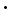The SDL Component Suite is an industry leading collection of components supporting scientific and engineering computing. Please visit the SDL Web site for more information....## CalcCenteredPolyFit

 Unit: SDL_math2 Class: Declaration:  function CalcCenteredPolyFit (const nOrder: integer; var kArray: TDoubleArray; var XShift: double; var FitQual: double; var NearSingular: boolean): boolean;  function CalcCenteredPolyFit (const nOrder: integer; var kArray: TDoubleArray; var alpha: TDoubleArray; var XShift: double; var FitQual: double; var NearSingular: boolean): boolean;

The method CalcCenteredPolyFit calculates the best fit for a polynomial of order nOrder which is shifted along the x-axis by XShift. If XShift is set to a zero value, the method automatically determines the optimal shift and returns it in XShift; any other value forces CalcCenteredPolyFit to use this value as shift. The regression equation of the shifted polynomial is given by

y = kArray + S(kArray[j](x-XShift) j)

The order of the polynomial is restricted to values between 1 and MaxPolyFitOrder. Higher orders may result in numerical instabilities. The variable array parameter kArray returns the polynomial coefficients, the variable parameter FitQual returns the goodness of fit. Version  of CalcCenteredPolyFit additionally calculates the p-values of the coefficients and returns them in the variable array alpha.

The function CalcCenteredPolyFit returns a TRUE value if the fit has been calculated successfully, and the returned parameters are valid. Numerical instabilities which may arise from near-singular equations are indicated by returning a TRUE value in the variable parameter NearSingular. In this case the calculated polynomial coefficients should not be used.

 Hint 1: The number of data used for the polynomial fit has to exceed the order of the polynomial at least by one.

 Hint 2: The open array parameter kArray has be resized to nOrder+1 before calling CalcCenteredPolyFit.

 Example: This method is used in the following example program (see http://www.lohninger.com/examples.html for downloading the code): curvefit

Last Update: 2017-Dez-21